# 几何表示（Geometry Representation）

## 隐式表面（Implicit Surface）

• 容易判断一个点是在几何体内部（$$f<0$$）还是外部（$$f>0$$​）。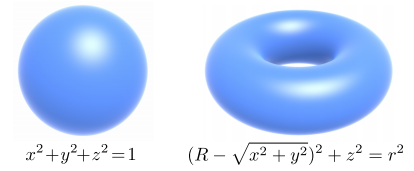## 显式表面（Explicit Surface）

• 相对容易采样，因为低维参数所需参数数量相对少了。
• 不容易判断点在几何体内部/外部（需要将多维参数解算成低维参数，这一步相当麻烦）。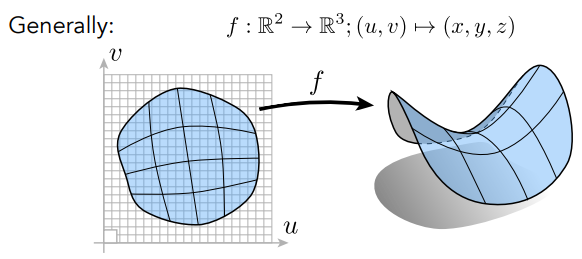## Constructive Soild Geometry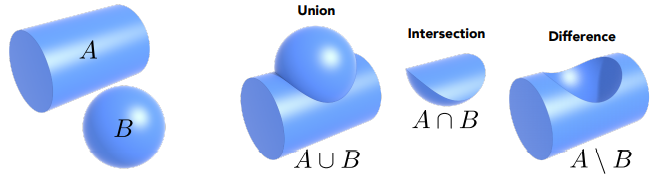## 有向距离场（Signed Distance Field,SDF）

• SDF 的插值会有着几何融合的效果（与一般的插值效果很不一样）：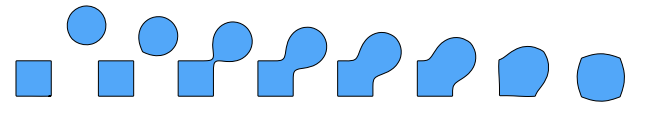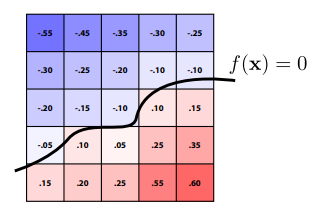## 点云（Point Cloud）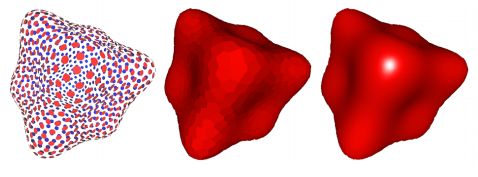## Polygon Mesh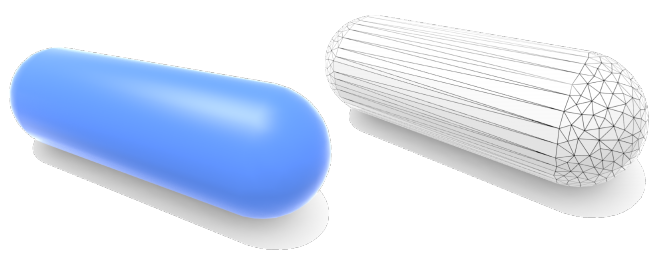# 射线相交（Ray Intersection）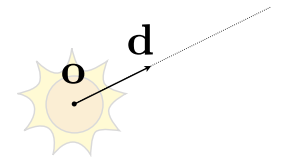$$f(\mathbf{o}+t \mathbf{d})=0$$

## 射线与球面相交$$(\mathbf{o}+t \mathbf{d}-\mathbf{c})^{2}-R^{2}= A t^{2}+B t+C=0$$

$$A=\mathbf{d} \cdot \mathbf{d}$$
$$B=2(\mathbf{o}-\mathbf{c}) \cdot \mathbf{d}$$
$$C=(\mathbf{o}-\mathbf{c}) \cdot(\mathbf{o}-\mathbf{c})-R^{2}$$

• $$B^2-4AC<0$$​​​​​ 时，无交点

• $$B^2-4AC=0$$ 时，有1个可能的交点，$$t=\frac{-B}{2 A}$$ （若交点不满足 $$0 \leq t<\infty$$，则不相交）

• $$B^2-4AC>0$$ 时，有2个可能的交点，$$t=\frac{-B \pm \sqrt{B^{2}-4 A C}}{2 A}$$ （若交点不满足 $$0 \leq t<\infty$$，则该交点不相交）

## 射线与平面相交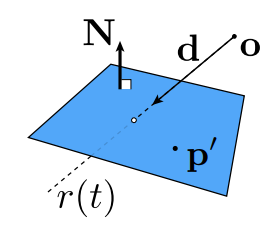$$\left(\mathbf{p}-\mathbf{p}^{\prime}\right) \cdot \mathbf{N}=\left(\mathbf{o}+t \mathbf{d}-\mathbf{p}^{\prime}\right) \cdot \mathbf{N}=0$$

• 有1个可能的交点， $$t=\frac{\left(\mathbf{p}^{\prime}-\mathbf{o}\right) \cdot \mathbf{N}}{\mathbf{d} \cdot \mathbf{N}}$$ （若交点不满足 $$0 \leq t<\infty$$，则不相交）

## 射线与三角形相交

1. 测试射线是否与三角形所在平面相交（若相交，将会得到相交点）
2. 测试相交点是否在三角形内部

## 射线与AABB相交

AABB：一个维度由两个标量表示，例如三维空间下的AABB应有 $$(x_0,x_1,y_0,y_1,z_0,z_1)$$​​ 来表示。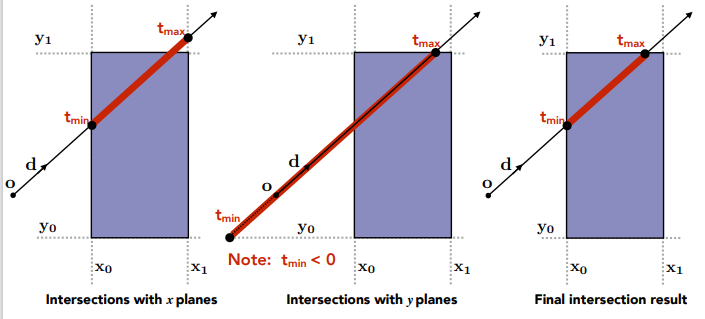$$t_{enter} = max \{t_{min}\}$$

$$t_{exit}=min\{t_{max}\}$$

• $$t_{exit} <0$$​​ ，无交点
• $$t_{exit}>=0$$$$t_{enter} < 0$$，意味着射线起点在AABB内部，拥有一个交点 $$t_{exit}$$
• $$t_{exit} >=0$$​ 且 $$t_{enter}<t_{exit}$$​​ ，两个交点分别为 $$t_{enter}$$$$t_{exit}$$

# 网格细分（Mesh Subdivision）

## Loop细分算法（Loop Subdivision）

Loop细分算法主要是针对基于三角形网格的细分。

1. 先将每个三角形拆分成四个小三角形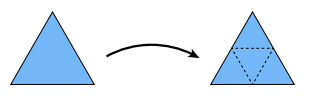1. 每个新顶点（由于三角形拆分而出现的）的位置应为：

$$V_{new} = \frac{3}{8} \cdot (A+B)+\frac{1}{8} \cdot (C+D)$$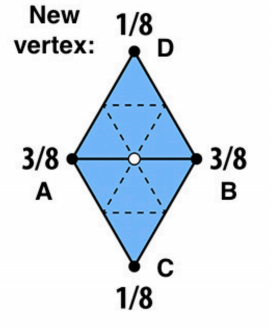1. 最后，每个旧顶点的位置也应该进行调整，应为：

$$V_{old}'=(1-n\cdot u) \cdot V_{old} + u \cdot \sum V_{neighbor}$$

$$u=\left\{\begin{array}{l}\frac{3}{16} \ ,n=3\\ \frac{3}{8n} \ ,otherwise \end{array}\right.$$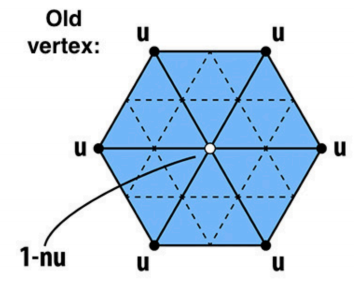Loop细分效果图：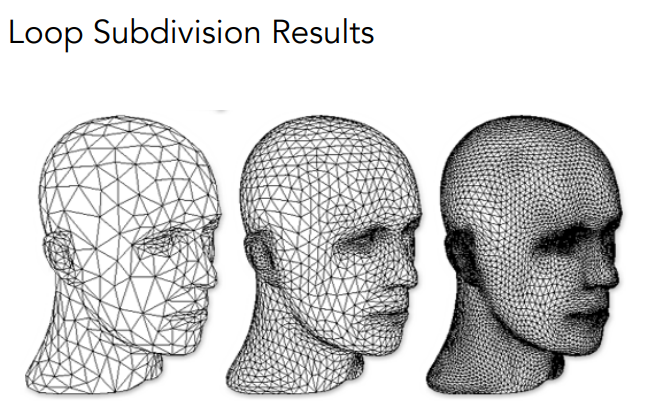## Catmull-Clack 细分算法（Catmull-Clack Subdivision）

Catmull-Clack 细分算法则是支持通用网格（如包含三角形、四边形）的细分，它的主要想法是每轮细分都在面的中心和边的中心生成新顶点，然后连接所有这些新顶点即可。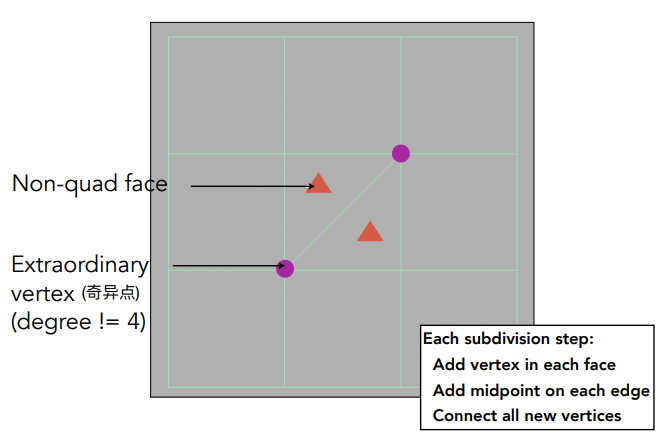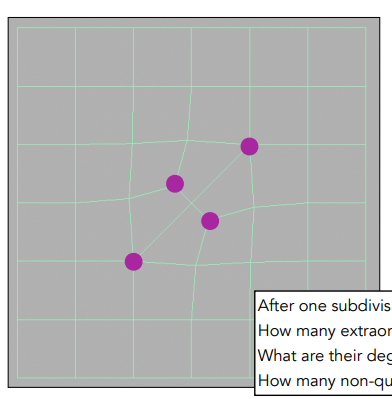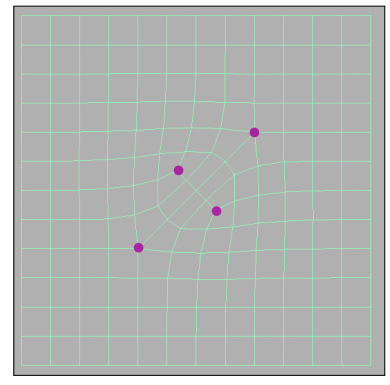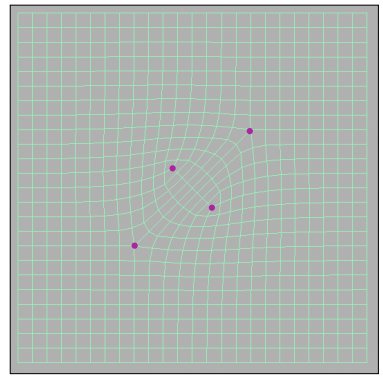1. 先生成每个面的Face point：

$$f=\frac{v_1+v_2+v_3+v_4}{4}$$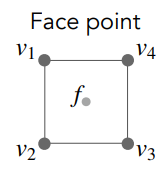1. 生成每个边的Edge point：

$$e=\frac{v_1+v_2+f_1+f_2}{4}$$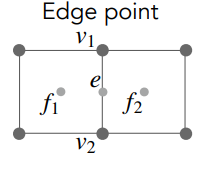1. 调整旧顶点的位置：

$$v=\frac{f_1+f_2+f_3+f_4+2(m_1+m_2+m_3+m_4)+4p}{16}$$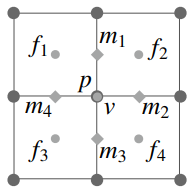Catmull-Clack 细分效果图：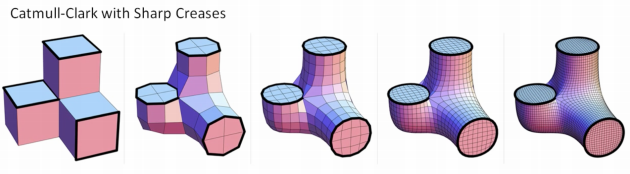## 曲面细分技术（Tessellation）

Tessellation 可被重新编程来用来实现如下技术（部分）：

• 网格细分（Mesh Subdivision）
• Displacement mapping
• 头发镶嵌
• 地形渲染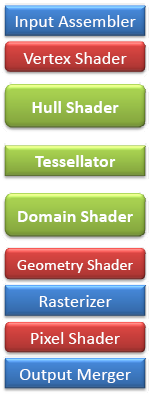# 网格简化（Mesh Simplification）

1. 网格简化最简单的想法是每次简化都坍缩（collapse）一条边，并且：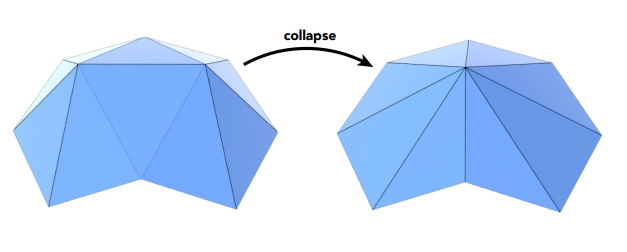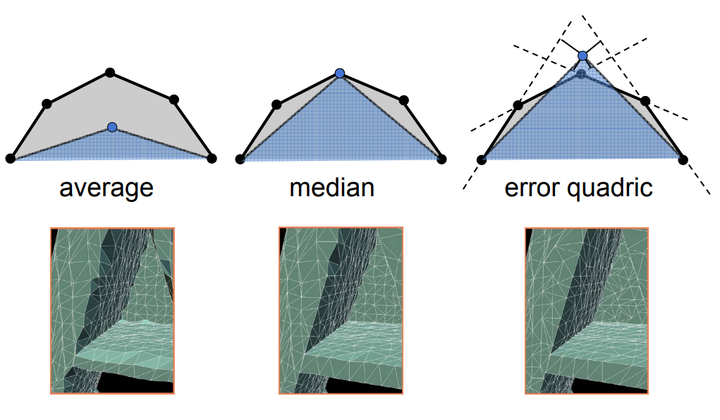1. 使用最小化 Quadric Error Metrics 方法（类比于机器学习里的最小二乘法）找到新顶点应当所在的位置即可。

## Level Of Details 技术（LOD）

LOD 技术：简单来说就是渲染远处模型的时候不一定要使用原始高精度的模型，而可以使用简化的模型来替代，从而减少提交GPU的顶点数。为了做到这种优化效果且避免低模穿帮，可以根据模型与摄像机的距离来决定模型的精度（或者说简化程度）。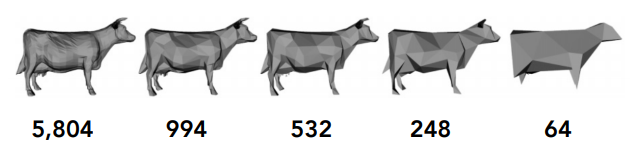LOD的思想也可以应用到游戏开发的其它方面，例如一些Component的update频率可以根据与摄像机的距离来适当减小，《刺客信条》的NPC基本上就是采用这种LOD方式，因为视野远处的NPC即使行为稍有些粗糙滞后都很难被察觉到。

# 参考

posted @ 2021-08-17 11:26  KillerAery  阅读(578)  评论(0编辑  收藏  举报